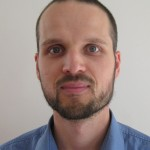# Manuel BodirskyManuel Bodirsky est chercheur au CNRS, en poste au Laboratoire d’Informatique de l’École Polytechnique. Il a passé sa thèse à l’Université Humboldt de Berlin. Son sujet principal est la complexité des problèmes de satisfaction de contraintes, et il s’interesse aux outils mathématiques de l’algèbre universelle, de la théorie des modèles, et de la combinatoire, qui peuvent être utilisés pour étudier ces problèmes et leurs complexité.

## Constraint Satisfaction: Finite Model Theory meets Infinite Model Theory

An important topic in finite model theory are logical characterizations of complexity classes. A paradigmatic example of such a characterization is Fagin’s theorem, which describes NP as the model-checking problem for existential second-order logic (ESO).

The question whether a there is a logical characterization for the class P, formalized by Gurevich, is an important open problem in finite model theory. One of the approaches to this problem is to design logics that capture larger and larger fragments of P (typically extensions of least fixed-point logic).

In this talk I present a different approach based on complexity classification for fragments of ESO. This will lead us to infinite-domain constraint satisfaction problems and questions from (infinite) model theory.

This entry was posted in Conférenciers invités. Bookmark the permalink.